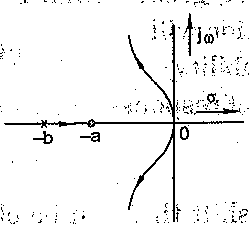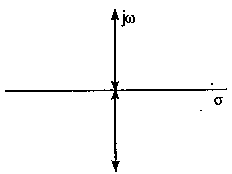# Which of the following points is NOT on the root locus of a system with the open-loop transfer function G(s)H(s)=K/ s(s + 1)(s + 3)

1.  s = -j√3,

2.  s =- 1.5

3.   s=- 3

4.  s=-∞

4

s =- 1.5

Explanation :
No Explanation available for this question

# The characteristic equation Of a feedback control system is given by s3 + 5s2 + (K +6) s + K = 0 where K > 0 is a scaler variable parameter. In the root-loci diagram ,of the: system, the, asymptotes of the root-locus four large values of K meet at a point in the s-plane, whose coordinates are

1.  (-3, 0)

2.  (- 2, 0)

3.  (- 1, 0)

4.  (2, 0)

4

(- 2, 0)

Explanation :
No Explanation available for this question

# A unity feedback System has an open-loop transfer ,function of the form KG(s)=K(s+a)/s2(s+b);b>a Which of the loci shown in the figure can be valid -loci for the system

1.

2.

3.

4.

4Explanation :
No Explanation available for this question

# A unity feedback system has an open loop transfer function, G(s) = K/s2 The root locus plot is

1.

2.

3.

4.

4Explanation :
No Explanation available for this question

# For a gain constant K, the phase-lead compensator

1.  reduces the slope of the magnitude curve in the entire range of frequency domain

2.  decreases the gain cross-over frequency

3.  reduce the phase margin

4.  reduce the resonance peak Mp.

4

reduces the slope of the magnitude curve in the entire range of frequency domain

Explanation :
No Explanation available for this question

# Consider the following statements Lead compensator 1. increases the margin of stability 2. speeds up transient response 3. does not affect the system error constant Of these statements

1.  2 and 3 are correct

2.  1 and 2 are correct

3.  1 and 3 are correct

4.  1, 2 and 3 are correct

4

1 and 2 are correct

Explanation :
No Explanation available for this question

# The maximum phase shift that can be provided by lead compensator with transfer function G(s)=1+6s/1+2s is

1.  15o

2.  30o

3.  45o

4.  60o

4

30o

Explanation :
No Explanation available for this question

# The transfer function of a compensating network is of the form (1+αTs)/(1+Ts).If this is a phase-lag network, the value of α should be

1.  exactly equal to 1

2.  between 0 and 1

3.  exactly equal to 1

4.  greater than 1

4

between 0 and 1

Explanation :
No Explanation available for this question

# It x is the system reactance and r is its resistance, the power transferred is maximum when

1.  x =r

2.  x =√2 r

3.  x=√3r

4.   x = 2r

4

x=√3r

Explanation :
No Explanation available for this question

# A phase-lag compensation will

1.  improve relative stability

2.  increase the speed of response

3.  increase bandwidth

4.  increase overshoot

4